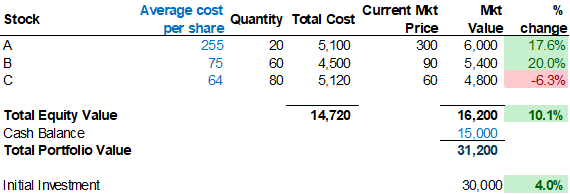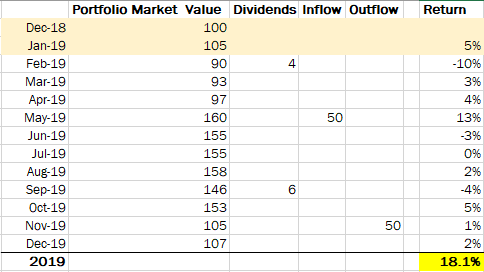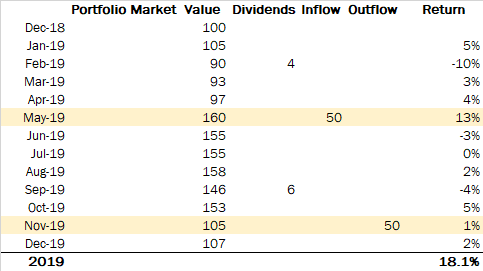### Calculating Portfolio Returns in 4 Easy Steps (Calculation File Included)

Posted on: 04 May, 2019

In our previous post, we discussed the importance of tracking portfolio returns meticulously. In this post, we will go over the actual methodology of calculating portfolio returns. To follow the examples shown in this post it is advisable to download the excel file that we have used.

#### Format of portfolio statementsReturn calculations start with the stock portfolio statements that brokers provide, usually on a daily basis. The image above shows an example of how a portfolio statement looks like in Bangladesh.

The initial investment made in this account is BDT30,000. The cost value and market value of individual stocks and the portfolio are shown (14,720 and 16,200 respectively). The cash remaining to be invested is reported below (15,000 in this case). The market value of the portfolio at the point of reporting is 31,200 (16,200+15,000).

Stock returns are calculated using the market value of portfolios. Therefore we must track this number to be able to perform our calculations. This is also known as Equity of the client and brokers generally report this number also. However, it will be wise to manually cross-check by adding stock value with cash as sometimes brokers report incorrect numbers.

There are some subtle differences in the format used by different stockbrokers. However by and large all of them report the things required for portfolio calculation. Let us now look at a simple example with a 4 step process in calculating returns.

#### Step 1: Decide on the return calculation periodWe need to decide in advance how frequently we want to calculate returns. Professional fund managers tend to calculate returns on a daily basis which gives the most accurate numbers. However, that also requires a lot of effort which is why we recommend most investors to calculate returns on a monthly basis.

Our example shown below will use monthly numbers for the calculation. For that, we need to record the portfolio market value for the portfolio at the end of each month. The return for a given month is simply the % change between the portfolio value of the two consecutive months.

For example, if the portfolio value on 31 December 2018 was BDT100 and value in 31st January 2019 was BDT105 then the return will be 105/100-1=5% for January.

#### Step 2: Deal with cash dividendsWhen companies give cash dividends the example above will be incorrect and some adjustment is needed. Due to dividends, cash goes out of the company balance sheet and goes to the investor’s bank account. That causes the stock price to come down which in turn reduces the market value of the portfolio to come down also.

Let us assume that the portfolio value was BDT 105 at the end of January and BDT 90 at the end of February. However, the investor received 4 as dividends during the month. In this situation, the correct return calculation will be (90+4)/105-1= -10%. We have added back the dividends to the market value of the portfolio to calculate the return for February.

#### Step 3: Adjust for inflows and outflowsSometimes we invest more in the portfolio while at other times we withdraw money from the portfolio. Inflows and outflows will artificially increase or decrease portfolio value and need to be adjusted also. In months where we have inflows, we need to deduct the inflow amount from the portfolio market value to calculate returns. For outflows, we do the opposite.

May 2019 Return: (160-50)/97-1= 13% (Inflow amount deducted) Nov 2019 Return: (105+50)/153-1=1%. (Outflow amount added back)

#### Step 4: Chain link the monthly returns to get annual returns

Finally to get to the 2019 full year return we need to chain link the monthly returns. The formula for chain linking is simple and shown below.

= (1+return(1))*(1+return(2)……*(1+return(n))-1

In our example the return for the year 2019 comes to 18.1%. There you have it. A correctly calculated time weighted return for the year.

We hope this post will allow you to start calculating your returns correctly from now on. Feel free to ask questions in the comments section if there is any confusion. We will come up with more posts in the future to address more advanced level topics in portfolio management.

• Tags:
• calculation, portfolio returns, 4 easy steps, portfolio statements, cash dividends, adjust, inflows, outflows, annual returns

#### Have Any Question?

If you have any questions feel free to reach out to us via phone or email.